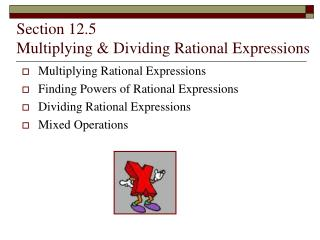DownloadDownload PresentationSection 12.5 Multiplying & Dividing Rational Expressions

# Section 12.5 Multiplying & Dividing Rational Expressions

Télécharger la présentation## Section 12.5 Multiplying & Dividing Rational Expressions

- - - - - - - - - - - - - - - - - - - - - - - - - - - E N D - - - - - - - - - - - - - - - - - - - - - - - - - - -
##### Presentation Transcript

1. Section 12.5 Multiplying & Dividing Rational Expressions • Multiplying Rational Expressions • Finding Powers of Rational Expressions • Dividing Rational Expressions • Mixed Operations

2. Example:Multiply Multiplying rational expressions works the same as multiplying fractions…you may multiply numerators and denominators and then simplify, or you can cross cancel common factors... With monomials, it’s a good idea to multiply first, and then simplify... Now reduce... 4 3 4y3 3

3. Multiplying Fractions (Parenthesize polynomials for clarity) To Simplify: Factor, then cancel like factors

4. Example – Step by Step x 1 1 4 1 1

5. Practice - Rational Multiplication

6. Practice - Rational Multiplication

7. Practice - Rational Multiplication

8. Dividing Fractions Change to multiplication by reciprocal, then follow the procedure for multiplication

9. Practice - Rational Division • Write down original problem1a. Rewrite as multiplication by reciprocal • Combine with parenthesized polynomials • Factor polynomials (if possible) • Rewrite (if any factoring was done) • Cancel out matching factors • Simplify the answer

10. Practice - Rational Division

11. Practice - Rational Division

12. Mixed Operations • Multiplications & Division are done left to right • In effect, make each divisor into a reciprocal

13. What Next? • Classwork/Homework